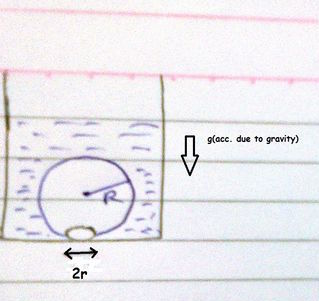# Surface tension of a water bubbleWhen we heat water in a vessel, air bubbles are formed at the bottom. These bubbles detach from the base and rise.

Take the bubbles to be spheres of radius $R$ and making a circular contact of radius $r$ with the bottom of the vessel. If $r < < R$, and the surface tension of water is $T$.

If the density of water is $\rho$, find the value of $r$ just before the bubbles detach.

×